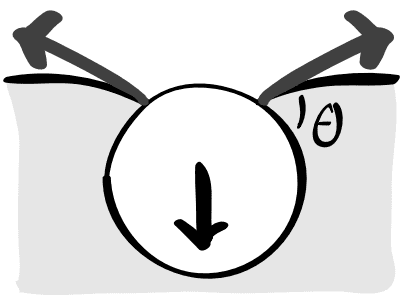# How Does Surface Tension Balance Small Objects on Water Surface?

• I
• Dario56

#### Dario56

When small object such as needle is put on the surface of water it displaces small amount of water which creates a depression under the object. Such depression increases surface area of the water because of which surface tension tends to decrease it.

Explanation why surface tension balances the weight of an object is that integration of surface tension along the contour or wetted length of the object yields that there is an resultant upward force balancing weight of an object if weight isn't bigger than that resultant. This upward resultant is essentially consequence of curved depression or contour under the object which I already mentioned.

Why is surface tension acting on the object since surface tension is a tendency to decrease surface area of the liquid because of which it should act on the liquid not the object?

There are both mechanical and thermodynamic approaches to this phenomena; a nice overview can be found here: https://arxiv.org/pdf/1211.3854.pdfThe resultant capillary forces [at angle ##\theta## to the pin surface], as well as the normal pressure forces [i.e. buoyant forces] acting everywhere at the surface, are balanced by the weight of the pin.

Are you asking why there is a capillary force exerted on the solid pin to begin with? Thermodynamically it arises due to the reduction in energy which results upon breaking up two liquid-liquid and solid-solid interfaces and then combining the resulting liquid-vacuum and solid-vacuum interfaces. See section C.2. of the aforementioned paper.

•vanhees71 and PeroK
There are both mechanical and thermodynamic approaches to this phenomena; a nice overview can be found here: https://arxiv.org/pdf/1211.3854.pdf

View attachment 290282

The resultant capillary forces [at angle ##\theta## to the pin surface], as well as the normal pressure forces [i.e. buoyant forces] acting everywhere at the surface, are balanced by the weight of the pin.

Are you asking why there is a capillary force exerted on the solid pin to begin with? Thermodynamically it arises due to the reduction in energy which results upon breaking up two liquid-liquid and solid-solid interfaces and then combining the resulting liquid-vacuum and solid-vacuum interfaces. See section C.2. of the aforementioned paper.
Yes, I am asking why is it that surface tension acts on the object when it should be a force acting on the liquid according to my understanding since surface tension tends to decrease surface area of the LIQUID NOT SOLID OBJECT.

Thanks for the paper. I did read some parts of this paper, but not part you mentioned.

Last edited:
Yes, I am asking why is it that surface tension acts on the object when it should be a force acting on the liquid according to my understanding since surface tension tends to decrease surface area of the LIQUID NOT SOLID OBJECT.

It's much more subtle than that! There are surface tension terms associated with interfaces of any phases, e.g. ##\gamma_{LS}## for liquid-solid, ##\gamma_{LV}## for liquid-vapour, etc., each defined as the derivative ##\dfrac{\partial E}{\partial A}## of free energy with respect to the area of that type of interface.

Capillary action arises because systems can lower their energy by extending certain interfaces at the expense of other interfaces!

•vanhees71, DaveE, PeroK and 1 other person
It's much more subtle than that! There are surface tension terms associated with interfaces of any phases, e.g. ##\gamma_{LS}## for liquid-solid, ##\gamma_{LV}## for liquid-vapour, etc., each defined as the derivative ##\dfrac{\partial E}{\partial A}## of free energy with respect to the area of that type of interface.

Capillary action arises because systems can lower their energy by extending certain interfaces at the expense of other interfaces!
Yes, this is true. So, you say it is actually surface tension on liquid - solid interface of an object that is responsible for force acting in the direction opposite of weight?

If this is so, how do techniques for measuring surface tension like Du - Nouy ring work since these methods are based exactly on force balance acting on some body on liquid surface (in this method ring made of platinum)? These methods are said to measure surface tension of the liquid not surface tension of liquid - solid interface.

If surface tension on liquid - solid interface balances weight of the ring than these methods should measure that surface tension, but as far as I know they don't because it is said that surface tension measured should be independent of solid body or material used.

•vanhees71
Again as is described in the paper, to separate a unit area of liquid-liquid interface (##E_1 = 2\gamma_{LV}##) and a unit area of solid-solid (##E_2 = 2\gamma_{SV}##) interface before recombining them into two unit area solid-liquid interfaces has an energy cost\begin{align*}
2\gamma_{LV} + 2\gamma_{SV} - 2A_{SL} \equiv 2\gamma_{SL}
\end{align*}where ##A_{SL}## is the energy reduction upon forming a solid-liquid interface. From Young's law,\begin{align*}
A_{SL} = (\gamma_{SV} - \gamma_{SL}) + \gamma_{LV} = \gamma_{LV} (\cos{\theta} + 1)
\end{align*}which is the force per unit length on the solid due to liquid-solid adhesion: this depends on ##\gamma_{LV}##.

Finally, to obtain the full picture for the capillary action, one must also take into account the normal Laplace forces acting on the pin, due to its curvature; these are of magnitude ##\kappa \gamma_{LV}## per unit length, where ##\kappa## is the curvature.

Last edited:
•vanhees71, hutchphd and PeroK